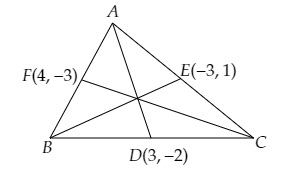# If the coordinates of the mid-points of the sides of a triangle be $(3, -2), (-3, 1)$ and $(4, -3)$, then find the coordinates of its vertices.

Given:

The coordinates of the mid-points of the sides of a triangle are $(3, -2), (-3, 1)$ and $(4, -3)$.

To do:

We have to find the coordinates of its vertices.

Solution:

Let $A (x_1, y_1), B (x_2, y_2)$ and $C (x_3, y_3)$ be the vertices of the $\triangle ABC$ and $D(3,-2), E(-3,1)$ and $F(4,-3)$ are the mid-points of $BC, CA$ and $AB$.$D$ is the mid-point of $BC$.

This implies,

$\frac{x_{2}+x_{3}}{2}=3$

$\Rightarrow x_{2}+x_{3}=6$.....(i)

$\frac{y_{2}+y_{3}}{2}=-2$

$\Rightarrow y_{2}+y_{3}=-4$......(a)

Similarly,

$E$ is the mid-point of $A C$.

$\frac{x_{3}+x_{1}}{2}=-3$

$\Rightarrow x_{3}+x_{1}=-6$.......(ii)

$\frac{y_{3}+y_{1}}{2}=1$

$\Rightarrow y_{3}+y_{1}=2$.......(b)

$\mathrm{F}$ is the mid-point of $\mathrm{AB}$.

$\frac{x_{2}+x_{1}}{2}=4$

$\Rightarrow x_{2}+x_{1}=8$........(iii)

$\frac{y_{2}+y_{1}}{2}=-3$

$\Rightarrow y_{2}+y_{1}=-6$.......(c)

Adding (i), (ii) and (iii), we get,

$2\left(x_{1}+x_{2}+x_{3}\right)=8$

$\Rightarrow x_{1}+x_{2}+x_{3}=4$......(iv)

Subtracting (i), (ii) and (iii) from (iv), we get,

$x_{1}=-2, x_{2}=10, x_{3}=-4$

Similarly,

Adding (a), (b) and (c), we get,

$2\left(y_{1}+y_{2}+y_{3}\right)=-8$

$\Rightarrow y_{1}+y_{2}+y_{3}=-4$.......(d)

Subtracting (a),(b) \) and (c) from (d), we get,

$y_{1}=0$ $y_{2}=-6$ $y_{3}=2$

Therefore, vertices of $\Delta \mathrm{ABC}$ are $\mathrm{A}(-2,0), \mathrm{B}(10,-6), \mathrm{C}(-4,2)$

The vertices of the triangle are $(-2,0), (10,-6)$ and $(-4,2)$.

Updated on: 10-Oct-2022

21 Views